# Sally

Sally is making 15 individual blueberry cheesecakes. How many pounds of blueberries will be in each cheesecake if 5 1/4 pounds of blueberries are divided equally among them?

x =  0.35 lb

### Step-by-step explanation: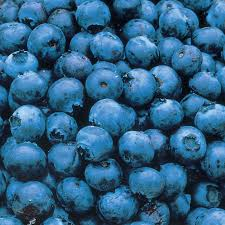Did you find an error or inaccuracy? Feel free to write us. Thank you!Tips to related online calculators
Need help to calculate sum, simplify or multiply fractions? Try our fraction calculator.

## Related math problems and questions:

• Dividing walnuts into crates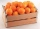There are 8 and 2 over 3 pounds of walnuts in a container, which will be divided equally into containers that hold 1 and 1 over 5 pounds. This would fill n and 4 over 18 containers. What is n?
• Land cost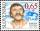The land is worth 33295 CZK. 1st owner has 5/15, 2nd owner has 4/15, 3rd owner has 1/5 4th owner has 1/5. How much CZK each of them will receive?
• Split between 4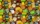There are 12 1/4 pounds of candy canes to split between four classes evenly. How many pounds of the candy canes will each class get?
• To improper fractionChange mixed number to improper fraction a) 1 2/15 b) -2 15/17
• Divide 11Divide the product of 4 and 5/8 by 1 1/2. Write your answer as a mixed number.
• How many 14How many 1/2 cup serving are in a package of cheese that contains 5 1/4 cups altogether?
• Numbers divisionWith what number should be divided mixed number 2 3/4 to get 11/12?
• Fractions and mixed numerals(a) Convert the following mixed numbers to improper fractions. i. 3 5/8 ii. 7 7/6 (b) Convert the following improper fraction to a mixed number. i. 13/4 ii. 78/5 (c) Simplify these fractions to their lowest terms. i. 36/42 ii. 27/45 2. evaluate the follow
• How many 17How many fifths are there in four wholes? How many 1/5 are there in 4?
• The sum 12The sum of 3 mixed numbers is 20 13/15. two of the numbers are 6 1/3 and 7 5/6. what is the third number?
• KarinaKarina has 5 4/7 meters of cloth. She needs 3 1/6 meters of it in making a curtain for her window. How many meters of cloth were left to her after making the curtain?
• A teacher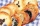A teacher baked 5 dozen cookies for the 16 students in her class. If the teacher divided the cookies equally among her students, how many cookies did she give each student?
• Reciprocals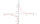Which among the given reciprocal is correct a. 3/15x1/3= 1 b. 3/20x20/3=1 c. 7/14x7/7=1 d. 34/3x34/34=1
• CherriesCherries in the bowl can be divided equally among 3 or 15 or 5 children. How many are the minimum cherries in the bowl?
• Rope cuttingShane has a piece of rope that is 7 4/5 units long. If he cuts it into pieces that are each 3/5 of a unit long, how many pieces does he have?
• A recipeA recipe requires 3/4 cups of milk. Paula is making 1/2 of the recipe. How many cups will Paula use?
• Evaluate expressionEvaluate expression using BODMAS rule: 1 1/4+1 1/5÷3/5-5/8